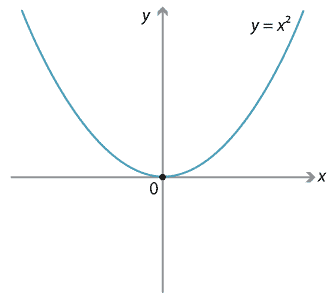## Content

### The basic parabola

The graph of the quadratic function $$y=x^2$$ is called a parabola. The basic shape can be seen by plotting a few points with $$x=-3$$, $$-2$$, $$-1$$, 0, 1, 2 and 3.Detailed description of diagram

We will refer to the above graph as the basic parabola. As we will see, all parabolas can be obtained from this one by translations, rotations, reflections and stretching.

The basic parabola has the following properties:

• It is symmetric about the $$y$$-axis, which is an axis of symmetry.
• The minimum value of $$y$$ occurs at the origin, which is a minimum turning point. It is also known as the vertex of the parabola.
• The arms of the parabola continue indefinitely.

Next page - Content - Transformations of the parabola